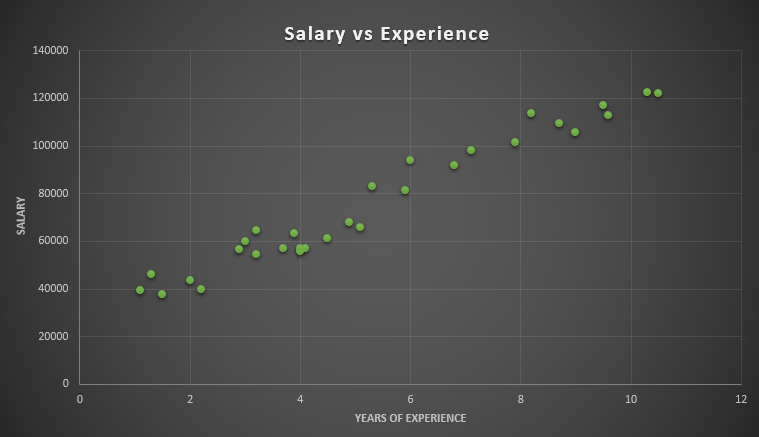Simple Linear Regression | Machine Learning

Simple Linear Regression: It is a linear regression model that uses two-dimensional sample points with one independent and one dependent variable(conventionally, the x and y coordinates). Then it tries to fit them in a linear function as follows-

y = b0 +b1x

With this function, a simple linear regression model will predict the dependent variable(denoted y) values as a function of the independent variables(denoted x). Basically, this function generates a straight line in the cartesian coordinate system. This straight line is the prediction line for the simple linear regression model which tries to predict the dependent values as accurately as possible.

Let's take an example of simple linear regression.

Suppose we have a dataset (Salary_Data.csv) that contains the salary of a certain number of employees according to their years of experience.

You can download the dataset from here.Let's plot the data in a graph.Here the plot shows the correlation between the Experience(in years) and Salary in our dataset. From this correlation, a simple linear regression model will try to find a straight line that best fits the data.

Now let's build a simple linear regression model with this dataset.

The first step will be preprocessing the dataset. As this model deals with just two variables, we can take the Experience column as the independent variable in the feature matrix X, and the Salary column as a dependent variable in the dependent variable vector y.

#Simple Linear Regression
# Importing the essential libraries
import numpy as np
import matplotlib.pyplot as plt
import pandas as pd
# Importing the dataset
X = dataset.iloc[:, 0].values
y = dataset.iloc[:, 1].values

Now, we will split the dataset between training and test sets. The training set will contain two third of the data and the test set will have one-third of the data. You can try taking arbitrary size for training and test sets. But keep in mind that the size of your datasets will make the model predict different outcomes. So, try to split the dataset in a way that helps the model to predict the best outcome.

# Splitting the dataset into the Training set and Test set
from sklearn.model_selection import train_test_split
X_train, X_test, y_train, y_test = train_test_split(X, y, test_size = 1/3, random_state = 0)

Note: Here, random_state = 0 is used to ensure that when you run this code, the output will be the same as us. You could run your code perfectly without this parameter. Though the training data set and test data set may not look exactly like ours, still it will be fine.

After preprocessing the data, now we will build our simple linear regression model. To fit the model to our training set, we just need to use the linear regression class from the Scikit-Learn library. The code in Python is as follows:

# Fitting Simple Linear Regression to the Training set
from sklearn.linear_model import LinearRegression
regressor = LinearRegression()
regressor.fit(X_train, y_train)

Now we have come to the final part. Our model is ready and we can predict the outcome! The code for this is as follows:

# Predicting the Test set results
y_pred = regressor.predict(X_test)

To plot our regression model outcome, we need the following lines of code:

To visualize the training set results:

# Visualising the Training set results
plt.scatter(X_train, y_train, color = 'red')
plt.plot(X_train, regressor.predict(X_train), color = 'blue')
plt.title('Salary vs Experience (Training set)')
plt.xlabel('Years of Experience')
plt.ylabel('Salary')
plt.show()Here the graph represents the linear regression line(the blue straight line) for the training set data. The algorithm tries to find the best fit line for our dataset. So this line is the best fit line the algorithm could find for our data.

Now it is time to see how our model predicts on the test data:

# Visualising the Test set results
plt.scatter(X_test, y_test, color = 'red')
plt.plot(X_train, regressor.predict(X_train), color = 'blue')
plt.title('Salary vs Experience (Test set)')
plt.xlabel('Years of Experience')
plt.ylabel('Salary')
plt.show()The above graph shows the predictions made by our simple linear regression model. The red dots are the test data and the blue line represents the predictions made by our model. If you look at the graph, our model made some close prediction for our test data(the dots on the line). Though the prediction is no accurate for some values(the dots far from the line), still it is a good simple linear regression model.

This is the simplest of all the regression models. In the following articles, we will see other complex regression models.